# Decimal Place Value

Print Rate 0 stars Common Core
Lesson size:
Message preview:
Someone you know has shared lesson with you:

To play this lesson, click on the link below:

https://www.turtlediary.com/lesson/decimal-place-value.html

Hope you have a good experience with this site and recommend to your friends too.

Login to rate activities and track progress.
Login to rate activities and track progress.
Decimal Place Value and Understanding Decimals Expressed in Word:

Just like whole numbers (or integers), decimal numbers also have place value and a corresponding number word. The place value of decimal numbers also allows you to know the number word of a decimal number. In other words, the number word of a decimal comes from its place value.

Let's look at the decimal number 4.25 which we can also write as four and twenty five hundredths. This number has both whole and a fractional parts. The "and" represents the decimal point. It is made up of:

4 ones (4 x 1)
2 tenths (2 x 0.1)
5 hundredths (5 x 0.01)

For this you may want to refer to the place value chart below: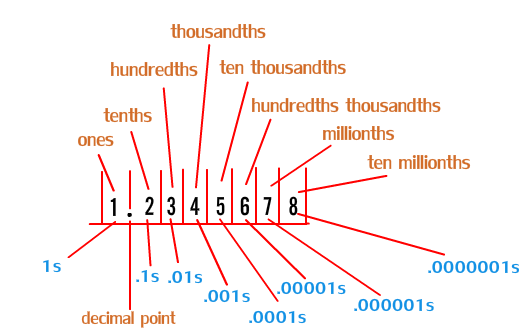First, let's look at some decimal numbers and see how they are expressed in words.

Example 1)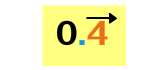0.4 is made up of 4 tenths (4 x 0.1)

The number word for 0.4 is four tenths and is the same as its place value.That is because the digit 4 is in the tenths place.

Remember, when dealing with decimals with the place value of tenths like 0.4 there are equivalent decimals to 0.4 such as to 0.40 (forty hundredths) and 0.400 (four thousandths), etc.

Example 2)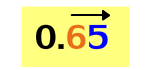and 0.65 is made up of 6 tenths (6 x 0.1)
5 hundredths (5 x 0.01)

The number word for 0.65 is sixty-five hundredths and is the same as its place value.

That is because the number 65 is to the right of the decimal point, indicating the decimal in the hundredths place.

Example 3)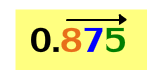and 0.875 is made up of 8 tenths (8 x 0.1)
7 hundredths (7 x .01)
5 thousandths (5 x 0.001)

The number word for 0.875 is eight hundred seventy-five thousandths.That is because the number 875 is to the right of the decimal point,indicating the decimal in the thousandths place.

Example 4)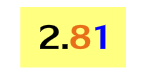and is made up of 2 ones (2 x 1)
8 tenths (8 x 0.1)
1 hundredths (1 x 0.01)

The number word for 2.81 is two and eighty-one hundredths.That is because there are 2 whole parts and 81 fractional parts. The "and" represents the decimal point.

Example 5)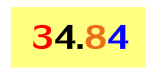and 34.24 is made up of 3 tens (3 x 10)
4 ones (4 x 1)
2 tenths (2 x 0.1)
4 hundredths(4 x 0.01)

The number word for 34.24 is thirty-four and twenty four hundredths.That is because there are 34 whole parts and 24 fractional parts. The "and" represents the decimal point.

Example 6)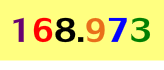and 168.973 is made up of 1 hundred (1 x 100)
6 tens (6 x 10) 8 ones (8 x 1)
9 tenths (9 x 0.1) 7 hundredths (7 x 0.01)

3 thousandths (3 x 0.001)

The number word for 168.973 is one hundred sixty-eight and nine hundred seventy-three thousandths.That is because there are 168 whole parts and 973 fractional parts. The "and" represents the decimal point.

Now let's look at number words and understand how they are expressed as decimals. Examine the chart below to see how the number word is expressed as a decimal:

Number Word
Decimal
three tenths
0.3
nine tenths
0.9
fifteen hundredths
0.15
forty-one hundredths
0.41
one hundred twenty-two thousandths
0.122
six hundred four thousandths
0.604
five and six tenths
5.6
sixteen and eight tenths
16.8
forty-one and fifty one hundredths
41.51
ninety and twelve thousandths
90.112
two hundred five and thirty-seven hundredths
205.37

As you can see, the number word simply expresses each decimal as its place value.

Notice how the word "and" is only used when there are both whole numbers and fractional parts. The word "and" shows there is a fractional part: it represents the decimal point.

Let's practice identifying the place value of decimal numbers and their number words.

1) What is the number word for the decimal 0.6?

Look at how many digits are to the right of the decimal. There is only one digit to the right of the decimal. The 6 is the only digit to the right, so the decimal is in the tenths place. Write the number 6 as a word (six) and then the word tenths.

2) What is the number word for the decimal 0.75?

Look at how many digits are to the right of the decimal. There are two digits to the right of the decimal. Two digits (7 and 5) are to the right of the decimal, making the decimal in the hundredths place. Write the number 75 as a word (seventy-five) and then the word hundredths.

3) What is the number word for the decimal 0.316?

Look at how many digits are to the right of the decimal. There are three digits to the right of the decimal. Three digits (3, 1 and 6) to the right of the decimal make the decimal in the thousandths place. Write the number 316 as a word (three hundred sixteen) and then the word thousandths.

4) What is the number word for the decimal 5.16?

Look at how many digits are to the left and right of the decimal. There is one digit to the left and two digits to the right of the decimal. The digit 5 is to the left indicating it has 5 ones. Two digits (1 and 6) to the right of the decimal make the fractional portion in the hundredths place. Write the number 5 as a word (five), then write the word and which represents the decimal point. To the right of the decimal point, write the number word for 16 (sixteen) and the word hundredths.

5) What is the number word for the decimal 21.745?

Look at how many digits are to the left and right of the decimal. There are two digits to the left and three digits to the right of the decimal. The digits 2 and 1 is to the left indicating it has 2 tens and 1 ones, or the number 21. Three digits (7, 4 and 5) to the right of the decimal make the fractional portion in the thousandths place. Write the number 21 as a word (twenty-one), then write the word and which represents the decimal point. To the right of the decimal point, write the number word for 745 (seven hundred forty-five) the word thousandths.

Answer: twenty-one and seven hundred forty-five thousandths

6) What is the number word for the decimal 609.4?

Look at how many digits are to the left and right of the decimal. There are three digits to the left and one digit to the right of the decimal. Three digits (6, 0 and 9) to the left represent the whole number 609. The one digit to the right (4) of the decimal make the fractional portion in the tenths place. Write the number 609 as a word (six hundred nine), then write the word and which represents the decimal point. To the right of the decimal point, write the number word for 4 (four) and the word tenths.

Answer: twenty-one and seven hundred forty-five thousandths

7) What decimal number is the same as two tenths?

Since the place value is tenths, just write the word two as the number 2 to the right of a decimal point. There is no whole number (or integer), so place a zero to the left of the decimal point.

8) What decimal number is the same as twenty-seven hundredths?

Since the place value is hundredths, just write the word twenty-seven as the number 27 to the right of a decimal point. There is no whole number (or integer), so place a zero to the left of the decimal point.

9) What decimal number is the same as three hundred fifteen thousandths?

Since the place value is thousandths, just write the word three hundred fifteen as the number 315 to the right of a decimal point. There is no whole number (or integer), so place a zero to the left of the decimal point.

10) What decimal number is the same as six and two tenths?

This number word uses "and" and has both whole numbers and fractional parts. Write the word six as the number 6 then a decimal point to represent the "and". Next, the place value of decimal is tenths, so just write the word two as the number 2 to the right of a decimal point.

11) What decimal number is the same as fourteen and fifty-two hundredths?

This number word uses "and" and has both whole numbers and fractional parts. Write the word fourteen as the number 14 then a decimal point to represent the "and". Next, the place value of decimal is hundredths, so just write the word fifty-two as the number 52 to the right of a decimal point.

## Decimal Place Value

• Decimal numbers have place value and a corresponding number word. Knowing the place value of decimal numbers also allows you to also know the number word of a decimal number.
• The number word of a decimal corresponds to its place value.
• 0.4 is a decimal number with a place value of tenth, so the number word for 0.4 is four tenths.
• 0.56 is a decimal number with a place value of a hundredth, so the number word for 0.56 is fifty-six hundredths.
• 0.178 is a decimal number with a place value of a thousandth, so the number word for 0.178 is one hundred seventy-eight thousandths.
• The word "and" is only used when there are both whole numbers and fractional parts. The word "and" represents the decimal point.

## Similar Lessons

Become premium member to get unlimited access.